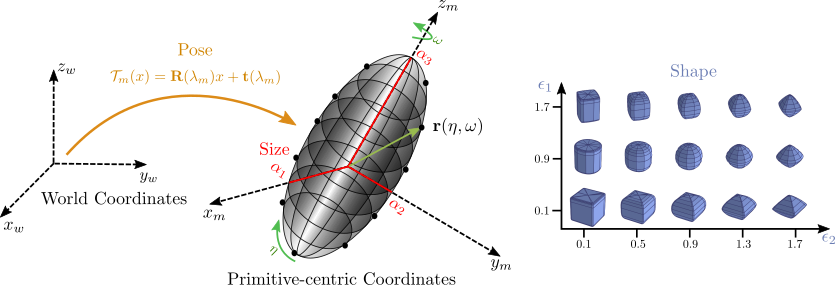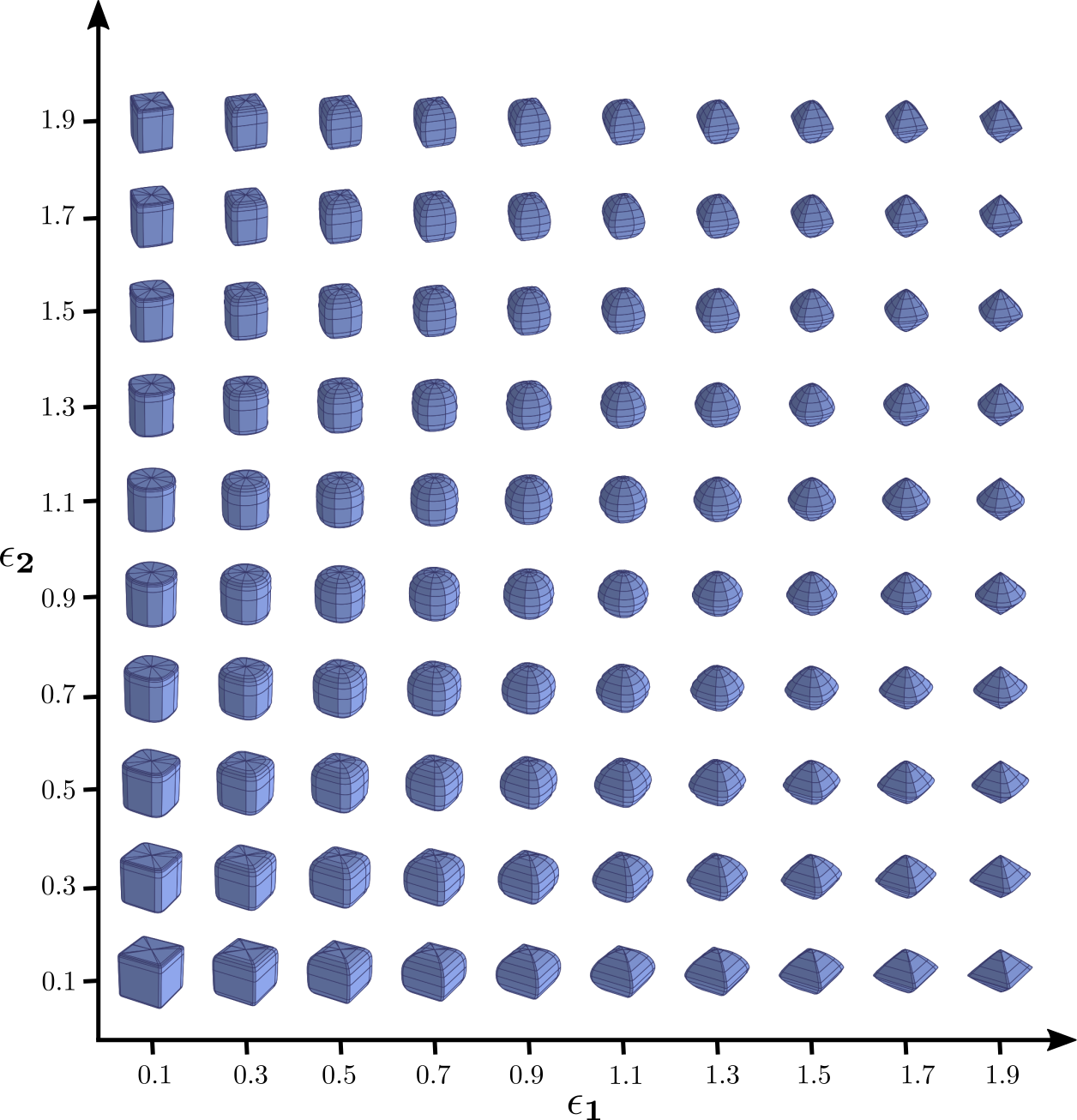Towards learning primitive-based representations

Humans develop a common-sense understanding of the physical behaviour of the world, within the first year of their life. For example, we are able to identify 3D objects in a scene and infer their geometric and physical properties, even when only parts of these objects are visible. It has long been hypothesized that the human visual system processes the vast amount of raw visual input into compact parsimonious representations, where complex objects are decomposed into a small number of atomic elements (primitives) that can each be represented using low-dimensional descriptions. In the early days of computer vision, researches explored various shape primitives that could potentially mimic the human's perception such as 3D polyhedrals, generalized cylinders, geons and superquadrics. However, it proved very difficult to extract such representations from images due to the lack of computational resources and training data at that time.

Recently, shape primitives have been revisited in the context of deep learning. Primitive-based representations provide an interpretable alternative towards traditional shape extraction methods that do not take into consideration the constituent parts of the target object.

@inproceedings{Paschalidou2019CVPR,
title = {Superquadrics Revisited: Learning 3D Shape Parsing beyond Cuboids},
author = {Paschalidou, Despoina and Ulusoy, Ali Osman and Geiger, Andreas},
booktitle = {Proceedings IEEE Conf. on Computer Vision and Pattern Recognition (CVPR)},
month = jun,
year = {2019},
}

@inproceedings{Paschalidou2020CVPR,
title = {Learning Unsupervised Hierarhical Part Decomposition of 3D Objects from a Single RGB Image},
author = {Paschalidou, Despoina and Luc van Gool and Geiger, Andreas},
booktitle = {Proceedings IEEE Conf. on Computer Vision and Pattern Recognition (CVPR)},
month = jun,
year = {2020},
}


Inspired by the nature of the human’s cognitive system, that perceives an object as a decomposition of parts, researchers have proposed to represent objects as a set of atomic elements, which we refer to as primitives. Examples for such primitives include 3D polyhedral shapes, generalized cylinders and geons for decomposing 3D objects into a set of parts. In 1986, Pentland introduced a parametric version of generalized cylinders, based on deformable superquadrics. He proposed a system able to represent the scene structure using multiple superquadrics.

Superquadrics are a parametric family of surfaces that can describe cubes, cylinders, spheres, ellipsoids etc in a single continuous parameter space. They are fully described using 11 parameters: 6 for the pose, 3 for the size and 2 for the shape. One of the most important features of superquadric surfaces is their interchangeable implicit and explicit function definition. The explicit superquadric function defines the surface vector $$\mathbf{r}$$that can be used for sampling points on the superquadric surface \begin{equation} \mathbf{r}(\eta, \omega) = \begin{bmatrix} \alpha_{1}\cos^{\epsilon_{1}}\eta \cos^{\epsilon_{2}}\omega \\ \alpha_{2}\cos^{\epsilon_{1}}\eta \sin^{\epsilon_{2}}\omega \\ \alpha_{3}\sin^{\epsilon_{1}}\eta \end{bmatrix} \quad \begin{aligned} -\pi/2 &\leq \eta \leq \pi/2\\ -\pi &\leq \omega \leq \pi \end{aligned} \label{eq:parametric_eq_supp} \end{equation}where $$\mathbf{\alpha} = [\alpha_{1}, \alpha_{2}, \alpha_{3}]$$ determine the size and $$\mathbf{\epsilon} = [\epsilon_{1}, \epsilon_{2}]$$ determine the global shape of the superquadric. Below we visualize the shape of superquadrics for different values of $$\epsilon_{1}$$ and $$\epsilon_{2}$$. In addition to the shape parameters, each superquadric is associated with a rigid body transformation. This transformation is represented by a translation vector $$\mathbf{t} = [t_{x}, t_{y}, t_{z}]$$ and a quaternion $$\mathbf{q} = [q_{0}, q_{1}, q_{2}, q_{3}]$$ that determines the coordinate system transformation from world coordinates to local primitive-centric coordinates.The implicit function can be used to decide the relative position of any 3D point w.r.t the superquadric surface. In particular, for any point $$\mathbf{x} \in \mathbb{R}^3$$, we can determine whether it lies inside or outside a superquadric using its implicit surface function which is commonly referred to as the inside-outside function. $$\begin{equation} f(\mathbf{x}; \lambda) = \left(\left(\frac{x}{\alpha_{1}}\right)^{\frac{2}{\epsilon_{2}}} + \left(\frac{y}{\alpha_{2}}\right)^{\frac{2}{\epsilon_{2}}}\right)^{\frac{\epsilon_{2}}{\epsilon_{1}}} + \left(\frac{z}{\alpha_{3}}\right)^{\frac{2}{\epsilon_{1}}} \label{eq:implicit_sq} \end{equation}$$ If $$f(\mathbf{x}; \lambda) = 1.0$$, $$\mathbf{x}$$ lies on the surface of the superquadric, if $$f(\mathbf{x}; \lambda) < 1.0$$ the corresponding point lies inside and if $$f(\mathbf{x}; \lambda) > 1.0$$ the point lies outside the superquadric.

We provide an easy-to-use script that can be used for visualizing the superquadric surface given a set of parameters. Code is available here.

References
• Peter Elias and Lawrence G Roberts. Machine perception of three-dimensional solids. PhD thesis, Massachusetts Institute of Technology, 1963
• I Binford. Visual perception by computer. In IEEE Conference of Systems and Control, 1971
• Alan H Barr. Superquadrics and angle-preserving transformations. IEEE Computer Graphics and Applications (CGA), 1981
• Irving Biederman. Recognition-by-components: a theory of human image understanding. Psychological Review, 94(2):115, 1987
• Ales Jaklic, Ales Leonardis, and Franc Solina. Segmentation and Recovery of Superquadrics, volume 20 of Computational Imaging and Vision. Springer, 2000